# Localization in Wireless Sensor Networks Using DV-Hop Algorithm and Fruit Fly Meta-heuristic

Localization in Wireless Sensor Networks Using DV-Hop Algorithm and Fruit Fly Meta-heuristic
Faculty of sciences, University of Ferhat ABBAS-SETIF 1, Setif 19000, Algeria

CS Department, Faculty of sciences and technology, University of adrar, Adrar 01000, Algeria

Institutes of Optics and Precision Mechanics (IOMP), University of Setif, Setif 19000, Algeria

Corresponding Author Email:
rabhi_seddik@yahoo.fr
Page:
18-23
|
DOI:
https://doi.org/10.18280/ama_b.620103
28 October 2018
|
Accepted:
3 February 2019
|
Published:
31 March 2019
| Citation

OPEN ACCESS

Abstract:

The sensor localization is one of many challenges in wireless sensor networks (WSNs). The perfect usage of WSNs largely depends on an efficient nodes’ localization system. This paper proposed an improved localization algorithm in WSNs called DV-hop (FOA) that is based on the classical algorithm DV-Hop (Distance Vector-Hop) and the Fruit Fly meta-heuristic to minimize the error on the nodes’ position estimation. Fruit fly optimization (FOA) is preferment for the minimization problems due to its quick convergence and less of parameters. In this work, we initialize the flies in the initial search location defined by the DV-hop method and they are given the random value of direction and distance. Then, we find out the flies with the highest smell value using fitness and keep its positions, and we consider it the location of the target node. Simulation results in matlab show that the proposed algorithm DV-hop (FOA) has the better localization error than the original DV-Hop algorithm.

Keywords:

WSN, FOA, DV-Hop, fruit fly, localization error, optimization, MATLAB

1. Introduction

The interest of research for Wireless Sensor Networks (WSNs) has increased with the potential for dependability, precision, suppleness, low cost and simplicity of deployment of these networks. A large majority of applications in WSNs use a random deployment of a big number of sensors, due either to the hostility of the area to be monitored or its immensity. The localization phase is therefore necessary not only for the operation of the network (geographical routing for example), but also for the exploitation of the collected data. It is therefore necessary to locate, with the best possible accuracy, all the nodes of the network [1-2]. This problem, despite the numerous research studies that have been carried out, remains an open issue.

Localization algorithms can be divided in range-based and range-free algorithms . A step prior to any range-based algorithm is to estimate the distances between nodes of the network and to determine the location using geometric methods (e.g. triangulation). This step uses some measurement techniques such as: ToA (Time of Arrival) , TDoA (Time Difference on Arrival) , AoA (Angle of Arrival)  and RSSI (Received Signal Strength Indicator) [9, 14]. The range-free methods are based on the connectivity information, such as DV-Hop algorithm [7, 10], APIT algorithm  and Centroid algorithms (e.g. MDS-MAP, CCA-MAP algorithms) [11-12].

DV-Hop algorithm finds node’s location based on the information of connectivity (hop count) between beacon (anchor) nodes and the target (unknown) nodes . This algorithm remains one of the most used algorithms because of their simplicity and practicability for sensor localization. However, the error between real position and the estimated position obtained by this algorithm needs to be reduced since it falls into the local minimum; several papers are published in order to address the latter [15-17]. In the last thirty years, there has been a growing trend in the number of studies conducted on animals living in groups or in society, and more particularly social insects. These studies in the theory of self-organization have given many researchers inspiration to develop a new approach called meta-heuristics. Meta-heuristics are general optimization algorithms applicable to a wide variety of problems. They appeared in order to solve many optimization problems. They are generally inspired from nature: biology (genetic algorithm, immune system, etc.) [18, 19], ethology (ant colony, Firefly etc.) [20, 21]. the application of meta-heuristics in the WSNs localization has known a particular evolution in recent years thanks to technological advances in machine calculation.

One of the innovative bio-inspired methods is the Fruit Fly optimization algorithm (FOA) developed in 2011 . In this paper, we use FOA to develop an efficient algorithm for localization in wireless sensor networks. The main idea is to use the DV-Hop method for estimating initial positions at the first step, and applied Fruit Fly optimization algorithm (FOA) at the second step to accurate the estimation error. The summation of the squared range error between the target node and the beacons nodes (the anchors) is used as the fitness to be minimized in this concept.

The rest of the paper is organized as follows: The next section provides reviews of the related works. In the section 3, the DV-Hop and the Fruit Fly algorithms used in this work are presented. Section 4 presents, the mathematical model of the localization problem and the steps of the proposed algorithm DV-Hop (FOA). Simulation results and localization performance analysis are given in Section 5. The conclusion of the paper is stated in section 6.

2. Related Work

Recently, there has been many meta-heuristics used in order to enhance the accuracy of the localization algorithms in wireless sensor networks. This section briefly exhibits several works published in the literature in this context.

In , authors used the meta-heuristic of simulated annealing to produce a localization algorithm called SAL, to determine the location of the unknown nodes in a randomly distributed sensor network. The simulations results have shown that SAL gives better accuracy than the semi definite programming localization . As in the most existing techniques of optimization, the SAL method also suffers from local minimum.

The work presented by Gopakumar and Jacob , based on Particle Swarm Optimization algorithm to diversify the search for the locations of the non-anchors nodes. The main idea of PSO localization algorithm is: randomly placed a group of particles in the research area; the local optimum is initialized with the value of particle positions; the particle with the best fitness value is considered as a global optimum; update velocity and position respectively and repeated this until the maximum number of generation are achieved. The performance of the PSO localization is compared and evaluated with the simulated annealing based WSN localization. Simulation results in work of Gopakumar and Jacob  shown that PSO has a better performance than SAL. However, this method needs a large number of anchors to localize all the unknown nodes.

Nan and al.  developed another localization approach based on genetic algorithm. This last defines positions of all unknown sensors by calculating their distances from all one-hop neighbors, simulation results shown that the approach minimizes the use of anchors compared to previous work. Recently, improved approaches based in Bees optimization algorithm, Firefly and Cuckoo Search algorithms have been published [27-29].

Marks and Niewiadomska-Szynkiewicz , propose a two-phase localization approach that uses a combination of genetic algorithm in the first phase and simulated annealing algorithm in the second one. This bio-inspired based scheme treated the flip ambiguity problem of localization.

In our work we propose a new hybrid approach, firstly uses DV-hop algorithm to obtain the unknown nodes initial coordinates. Secondly, it adopts fruit fly Optimization (FOA) algorithm to obtain the unknown nodes final coordinates. The FOA bio-inspired approach is used because it is recently invented to find locations in a multi-dimensional search space and to benefit from the advantages of meta-heuristics in order to improve the accuracy of a known classical method of localization.

3. Overview of Dv-Hop and Fruit Fly

3.1 DV-HOP algorithm

DV-hop is a distributed positioning algorithm that has been proposed by Dragos Niculescu and Badri Nath . In DV-Hop, three steps are considered to search the positions of unknown nodes, described as follows:

Step 1: initially all anchors diffuse their locations to the other nodes in the network. The messages are propagated hop by hop where a hop counter is included in the message. Each node maintains an information table on the anchor node and counts the minimum number of hops that separates it from each anchor.

Step 2: When an anchor node receives a message from another anchor node, it estimates the average distance in terms of hops using the locations of two anchors and the hop counter, and returns it to the network as a correction factor. An anchor with (xi, yi) coordinates, estimates the average distance (hop-size I) using the following equation:

HopSize$_{i}=\frac{\sum_{i \neq j} \sqrt{\left(x_{i}-x_{j}\right)^{2}+\left(y_{i}-y_{j}\right)^{2}}}{\sum_{i \neq j} h_{i j}}$       (1)

where (xj, yj) are the coordinates of the anchor j, h i j is the number of hops separating the anchor i from the anchor j. Then, after that all the unknown nodes have received the hop-size from the anchor nodes, they compute the distance (di) to the anchor nodes based on the hop-sizei and hid (the minimum hop count), using the following equation:

$d_{i}=h_{id}×HopSize_i$        (2)

Step 3: A multilateration method is used to calculate the unknown node’s position according to the distance to each anchor node obtained in the step 2. Let (xi, yi) be the coordinates of the anchor i, the unknown node location X (x, y) T can be found using the following formula:

$\left\{\begin{array}{l}{\left(x_{1}-x\right)^{2}+\left(y_{1}-y\right)^{2}=d_{1}^{2}} \\ {\left(x_{2}-x\right)^{2}+\left(y_{2}-y\right)^{2}=d_{2}^{2}} \\ {\ldots} \\ {\left(x_{n}-x\right)^{2}+\left(y_{n}-y\right)^{2}=d_{n}^{2}}\end{array}\right.$     (3)

Eq. (3) can be written in the following linear equation:

AX = b       (4)

where:

$A=-2 \times\left[\begin{array}{cc}{x_{1}-x_{n}} & {y_{1}-y_{n}} \\ {x_{2}-x_{n}} & {y_{2}-y_{n}} \\ {\cdots} & {} \\ {x_{n-1}-x_{n}} & {y_{n-1}-y_{n}}\end{array}\right]$       (5)

$b=\left[\begin{array}{c}{x_{1}^{2}-x_{n}^{2}+y_{1}^{2}-y_{n}^{2}+d_{n}^{2}-d_{1}^{2}} \\ {x_{2}^{2}-x_{n}^{2}+y_{2}^{2}-y_{n}^{2}+d_{n}^{2}-d_{2}^{2}} \\ {\ldots} \\ {x_{n-1}^{2}-x_{n}^{2}+y_{n-1}^{2}-y_{n}^{2}+d_{n}^{2}-d_{n-1}^{2}}\end{array}\right]$     (6)

$X=\left[\begin{array}{l}{x} \\ {y}\end{array}\right]$        (7)

The least-squares estimate of X is:

$X=\left(A^{T} A\right)^{-1} A^{T} B$   (8)

3.2 Fruit fly optimization algorithm

Fruit Fly Optimization is an evolutionary computation algorithm invented by Prof. Wen-Tsao Pan, a scholar of Taiwan in 2011 . It is a new meta-heuristic method for solving global optimization based on the food finding behavior of drosophila (fruit fly species). Compared to other intelligent techniques, fruit fly optimization algorithm (FOA) is easy to implement and has less parameter (number of flies and maximum generations only).

In nature, for searching the food, drosophila especially uses the sense of smell and vision of the sensory perception. This insect has an osphresis organ that can sniff food 40 km away. In the idea inspired from the Drosophila’s biological behavior, the population is invariant and to find the food, the Fruit Fly estimates the concentration of the food taste by a smell concentration judgment function. Then, she flies by vision to the Fruit Fly nearest to the food. Every time, she estimates and gets the best position of the Fruit Fly where the smell concentration is the highest.

According to the features of the fruit fly group, the FOA's main steps are described as follows:

Step 1: Initialize the place of the first Fruit Fly group’s randomly, as shown in Figure 1.

Init (X_axis,Y_axis);

Step 2: Search the direction of the food using the osphresis of a fruit fly individually, by giving the random value of the direction and the distance.

Xi = X_axis+RandomValue();

Yi = Y_axis+RandomValue();

Where, i vary from 1 to the population size.

Step 3: Smell the concentration judgment value (Si) where is the reciprocal of the distance to the origin, and is calculated if the location of the food cannot be known

Distance$_{i}=\sqrt{X_{i}^{2}+Y_{i}^{2}} S_{i}=\frac{1}{\text { Distance }_{i}}$

## 1.jpg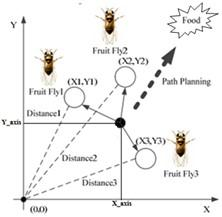Figure 1. Searching the food by the fruit fly group's

Step 4: Find the smell concentration (Smelli) of the individual fruit fly by substituting the value of Si into the smell concentration judgment function (Fitness).

Smelli = Function (Si)

Step 5: Determine the fruit fly with the optimum (maximum or minimum) smell concentration between the fruit fly group's.

[bestsmell bestindex]=optimal (smell)

Step 6: Fruit fly group's fly toward the best position of the food by vision where they maintain the best smell concentration value and the coordinates correspondingly.

Smellbest = bestSmell

X_axis = X (bestIndex)

Y_axis = Y (bestIndex)

Step 7: Enter into an iterative optimization to repeat the execution of the steps 2-5. If the smell concentration value is higher than the previous iterative value, then execute the step 6.

4. The Proposed Approach: Dv-Hop (Foa)

4.1 Problem formulation

In wireless sensor network, we assume that there are N sensors deployed randomly on a D-dimensional (D=2 in this work) space. The objective of localization is to estimate the coordinates of unknown nodes using a priori information about the location of M anchor sensors. The distance between the estimated position and the real position of nodes is the location errors given by the following equation:

Error$_{i}=\sqrt{\left(\hat{x}_{i}-x_{i}\right)^{2}+\left(\hat{y}_{i}-y_{i}\right)^{2}}$       (9)

4. Where $\left(\hat{x}_{i}, \hat{y}_{i}\right)$ the estimated coordinates of the sensor i and  $\left(x_{i}, y_{i}\right)$  its real coordinates i=(1,2…. N-M)

The most important criterion of the localization problem is the average error. In this paper, it is calculated by the following formula:

$\operatorname{Error}_{a v e}=\frac{1}{M} * \sum_{i=1}^{M} \operatorname{Error}_{i}$           (10)

The objective function of the FOA algorithm for the nodes’ localization problem can be calculated as:

$f(x, y)=\frac{1}{M} * \sum_{i=1}^{M}\left(\sqrt{\left(x-x_{i}\right)^{2}+\left(y-y_{i}\right)^{2}}-s\right)^{2}$  (11)

2. Where M ≥ 3 is the number of anchor nodes.

(xi,yi) is the coordinates of the ith anchor node

(x,y) is the coordinates of the Fruit Fly to be evaluated.

S is the smell concentration judgment value of the Fruit Fly to be evaluated.

Different affiliations shall be listed in separate lines. Do not insert any punctuation at the end of each affiliation. If all the authors are affiliated to the same organization, type that affiliation just once.

4.2 The steps of the improved algorithm

In order to solve the localization problem, the proposed algorithm executes DV-hop localization algorithm firstly to obtain the initial locations of nodes. Further, the algorithm initializes the Fruit Fly with such initial locations. The localization process is divided into the following three phases:

(1).            Initialization: N sensors with radius R are deployed Randomly alongside to M anchors in a 2D field; then, we determine the number of fruit fly group's (SizPop) and the maximum generation (MaxGen) used for the FOA algorithm.

(2).            Initial search: In this phase, we use DV-hop method to estimate the initial locations of the unknown nodes. For this purpose, each anchor calculates and broadcasts the average distance per hop for all the nodes in the network, and every unknown node is localized by trilateration along with the anchor nodes. The positions obtained will be considered as the initial estimate locations of the unknown nodes.

(3).            Optimization: In this phase we apply the Fruit Fly optimization (FOA) method for minimizes the location error to obtain the relative coordinates of nodes as follows:

-          For every unknown node, we put N' (N'=SizPop) flies in their initial search location defined by the DV-hop method;

-          Gives the random value of direction and distance of each flies, finds out the flies with the highest smell value using the fitness and keeps its positions;

-          Repeat the process by comparing the previous positions with the current ones by keeping the best ones until the stop condition (MaxGen) is exhausted.

-          Consider the last obtained positions as the coordinates relative to such unknown node.

The detailed processing steps of the approach DV-Hop (FOA) are shown on the flowchart of the Figure 2.

## 2.jpg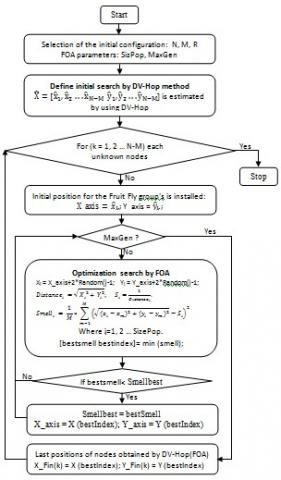Figure 2. Flowchart of DV-Hop(FOA) algorithm

5. Simulation Results

In this section, we use computer simulations in MATLAB platform to evaluate and to demonstrate the performance of the proposed algorithm DV-Hop (FOA). Then, we compare the proposed algorithm with the original DV-Hop algorithm in terms of location error and the average location error.

The experiment area is a square regular grid topology with a fixed size of 50*50 m2 and a transmission radius of sensor nodes (R) set to 20m. Figure 3 shows a simple example of the square network with N=50 nodes randomly spreading where M=10 are anchor nodes. Black points represent unknown nodes and red points represent anchor nodes. The parameters of the proposed algorithm are: Population=20 fruit fly and maximum generations =100.

## 3.jpg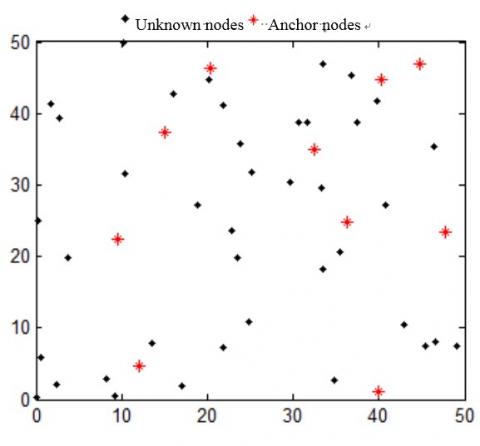Figure 3. Simulation example of WSN with 50 nodes randomly placed in 2-d field

## 4.jpg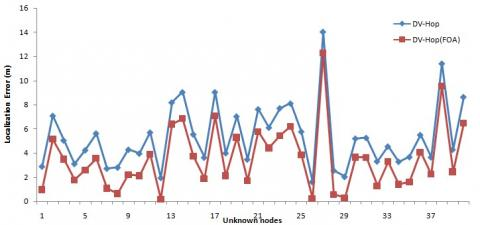Figure 4. Location error for each unknown node

Figure 4 shows the graph of the location error for each unknown node in the case of the DV-hop method and the DV-Hop (FOA) algorithm. These diagrams correspond to the configuration of the Figure 3. These results clearly show that the proposed approach minimizes the location error for each target node obtained by the DV-Hop algorithm. Therefore, DV-Hop (FOA) minimizes the average location error calculated by the original DV-hop as shown in the following table:

Table 1. Average error calculated by DV-Hop (FOA) and DV-Hop

 DV-Hop DV-Hop (FOA) average error of localization 5.35206 3.5504

In order to show the effect of changing the parameters, more intuitively, we analyze each characteristic independently. Firstly, we fixe all the parameters of the algorithms and we varied the number of nodes spread in the 2d field from 10 to 100. The obtained results are shown in Figure 5.

Secondly, we deploy randomly 50 sensors in the 2D space. We change the number of the anchor nodes between 5 and 20 nodes and we keep fixed all the parameters of the algorithms. Figure 6, illustrates the obtained results.

## 5.jpg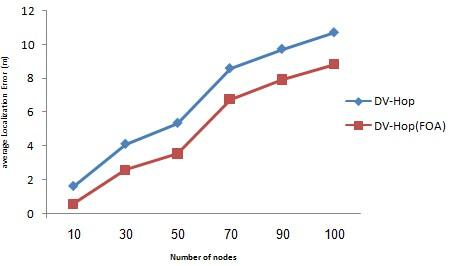Figure 5. Average localization error Vs number of nodes

## 6.jpg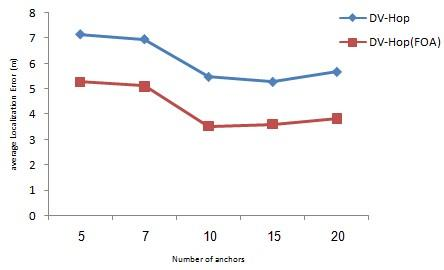Figure 6. Average localization error Vs number of anchors

The experimental results obtained in Figures 5 and 6 show for the two algorithms that the estimated average error grows when the number of sensors increases and decreases when the number of anchor nodes increases until 10 nodes. Then it stabilizes and increases slightly up to 20 anchors. Hence, in order to determine the location of any sensor we need, at least, for the distance between this latter and three anchors, so it is probably that we cannot get the location of some sensors when we use a few number of anchors.

Finally, we study the effect of changing the number of flies on the results obtained by the proposed method. For this, we use the parameters having given the best results in the previous study and we change the number of flies from 5 to 40. The obtained results show the effect of increasing the number of flies on the average error. The Figure 7 show that the use of 20 flies almost guarantees a good result. Further, when getting the sufficient number of flies proportional to the network configuration, we will get almost the same precision.

## 7.jpg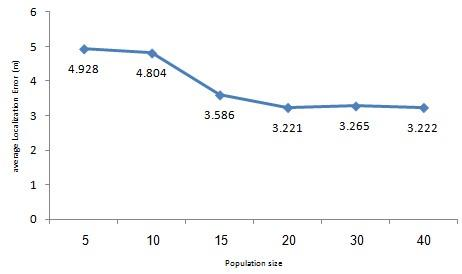Figure 7. Average localization error Vs population size

6. Conclusions

The localization in the WSNs is to find the locality (or position) of one or more sensors collected randomly in a multidimensional field to accomplish a specific task. Nowadays, the existing automatic locating systems are numerous and use several technological paths, they are very different from each other but generally respond to a common need (minimize the error between the real and the estimate position of each unknown sensors). The classical methods based on connectivity and/or geometry like DV-hop, DV-distance, APIT, ... and acceptable solutions to the problem of localization but the precision obtained by these algorithms always needs an optimization. Recently, meta-heuristics are widely used by researchers to deal with optimization problems.

In this paper, the fruit fly optimization algorithm is applied to minimize the localization error between real and estimated coordinates of the sensors. In the proposed approach DV-Hop (FOA), we initialize the fruit fly group's by the initial locations calculated by the DV-Hop method in a preliminary step. The simulation results show that the proposed algorithm provides a lower average localization error in comparison with the original DV-Hop algorithm.

In this work, we could show the ability of meta-heuristics in improving the accuracy of the localization in classical techniques. Therefore, we recommended that these methods could be used and hybridized in order to obtain better results. Moreover, the meta-heuristics are less complicated than traditional methods and thus, consume less energy. Our future objective is to apply the proposed approach in 3d field and propose a parallel version.

References

 Paul, A.K., Sato, T. (2017). Localization in wireless sensor networks: A survey on algorithms, measurement techniques, applications and challenges. Journal of Sensor and Actuator Networks, 6(4): 24. https://doi.org/10.3390/jsan6040024

 Srikanth, B., Kumar, H., Karanam, U.M., Rao, (2018). A robust approach for WSN localization for underground coal mine monitoring using improved RSSI technique. Mathematical Modelling of Engineering Problems, 5(3): 225-231. https://doi.org/10.18280/mmep.050314

 Girod, L., Bychkovskiy, V., Elson, J., Estrin, D. (2002). Locating tiny sensors in time and space: A case study. In Computer Design: VLSI in Computers and Processors, 2002. Proceedings. 2002 IEEE International Conference on IEEE, pp. 214-219. https://doi.org/10.1109/ICCD.2002.1106773

 Yu, K., Oppermann, I. (2004). Performance of UWB position estimation based on time-of-arrival measurements. In Ultra Wideband Systems, 2004. Joint with Conference on Ultrawideband Systems and Technologies. Joint UWBST & IWUWBS. 2004 International Workshop on IEEE, pp. 400-404. https://doi.org/10.1109/UWBST.2004.1321004

 Harter, A., Hopper, A., Steggles, P., Ward, A., Webster, P. (2002). The anatomy of a context-aware application. Wireless Networks, 8: 187-197. https://doi.org/10.1023/A:1013767926256

 Niculescu, D., Nath, B. (2003). Ad hoc positioning system (APS) using AOA. In INFOCOM 2003. Twenty-Second Annual Joint Conference of the IEEE Computer and Communications. IEEE Societies, 3: 1734-1743. https://doi.org/10.1109/INFCOM.2003.1209196

 Zhong, J.W.W.L. (2008). Study on the application of DV-Hop localization algorithms to random sensor networks. Journal of Electronics & Information Technology, 4(51). http://dx.doi.org/10.3724/SP.J.1146.2006.01511

 He, T., Huang, C., Blum, B.M., Stankovic, J.A., Abdelzaher, T. (2003). Range-free localization schemes for large scale sensor networks. In Proceedings of the 9th Annual International Conference on Mobile Computing and Networking ACM, pp. 81-95. https://doi.org/10.1145/938985.938995

 Bahl, P., Padmanabhan, V.N. (2000). RADAR: An in-building RF-based user location and tracking system. INFOCOM 2000. Nineteenth Annual Joint Conference of the IEEE Computer and Communications Societies. Proceedings. IEEE, 2: 775-784. https://doi.org/10.1109/INFCOM.2000.832252

 Niculescu, D., Nath, B. (2003). DV based positioning in ad hoc networks. Telecommunication Systems, 22(1-4): 267-280. https://doi.org/10.1023/A:1023403323460

 Ji, X., Zha, H. (2004). Sensor positioning in wireless ad-hoc sensor networks using multidimensional scaling. In INFOCOM 2004. Twenty-third Annual Joint Conference of the IEEE Computer and Communications Societies IEEE, 4: 2652-2661.https://doi.org/10.1109/INFCOM.2004.1354684

 Li, L., Kunz, T. (2007). Localization applying an efficient neural network mapping. In Proceedings of the 1st international conference on autonomic computing and communication systems (p. 1). ICST (Institute for Computer Sciences, Social-Informatics and Telecommunications Engineering). http://dx.doi.org/10.4108/ICST.AUTONOMICS2007.2126

 Boushaba, M., Hafid, A., Benslimane, A. (2009). High accuracy localization method using AoA in sensor networks. Computer Networks, 53(18): 3076-3088. http://dx.doi.org/10.1016/j.comnet.2009.07.015

 Cho, H.H., Lee, R.H., Park, J.G. (2011). Adaptive parameter estimation method for wireless localization using RSSI measurements. Journal of Electrical Engineering and Technology, 6(6): 883-887. http://dx.doi.org/10.1007/978-3-642-22309-9_29

 Yu, Y., Jiang, C., Zhao, X., Yun, L., Li, Z., Ren, Y. (2011). Sequence-based localization algorithm with improved correlation metric and dynamic centroid. Science China Information Sciences, 54(11): 2349. https://doi.org/10.1007/s11432-011-4434-1

 Ding, J., Zhang, L., Cheng, G., Ling, Z., Zhang, Z., Lei, Y. (2012). Study on DV-Hop algorithm based on modifying hop count for wireless sensor networks. International Journal of Computer Science Engineering & Technology, 2(10): 452-1456.

 Sassi, H., Najeh, T., Liouane, N. (2014). A selective 3-anchor DV-Hop algorithm based on the nearest anchor for wireless sensor network. World Academy of Science, Engineering and Technology, International Journal of Computer, Electrical, Automation, Control and Information Engineering, 8(10): 1756-1760.

 Fonseca, C.M., Fleming, P.J. (1993). Genetic algorithms for multiobjective optimization: Formulation discussion and generalization. In Icga, 97(7): 416-423.

 Hofmeyr, S.A., Forrest, S. (2000). Architecture for an artificial immune system. Evolutionary Computation, 8(4): 443-473. https://doi.org/10.1162/106365600568257

 Dorigo, M., Di Caro, G. (1999). Ant colony optimization: a new meta-heuristic. In Evolutionary Computation, 1999. CEC 99. Proceedings of the 1999 Congress on IEEE, 2: 1470-1477. https://doi.org/10.1109/CEC.1999.782657

 Yang, X.S. (2009). Firefly algorithms for multimodal optimization. In International Symposium on Stochastic Algorithms, Springer, Berlin, Heidelberg, 169-178. https://doi.org/10.1007/978-3-642-04944-6_14

 Pan, W.T. (2012). A new fruit fly optimization algorithm: taking the financial distress model as an example. Knowledge-Based Systems, 26: 69-74. https://doi.org/10.1016/j.knosys.2011.07.001

 Kannan, A.A., Mao, G., Vucetic, B. (2006). Simulated annealing based wireless sensor network localization. JCP, 1(2): 15-22. https://doi.org/10.1109/VETECS.2006.1682979

 Biswas, P., Ye, Y. (2004). Semidefinite programming for ad hoc wireless sensor network localization. In Proceedings of the 3rd international symposium on Information processing in sensor networks ACM, 46-54. https://doi.org/10.1109/IPSN.2004.1307322

 Gopakumar, A., Jacob, L. (2008). Localization in wireless sensor networks using particle swarm optimization. IET International Conference on Wireless, Mobile and Multimedia Networks. https://doi.org/10.1049/cp:20080185

 Nan, G.F., Li, M.Q., Li, J. (2007). Estimation of node localization with a real-coded genetic algorithm in WSNs. In Machine Learning and Cybernetics, 2007 International Conference on IEEE, 2: 873-878. https://doi.org/10.1109/ICMLC.2007.4370265

 Moussa, A., El-Sheimy, N. (2010). Localization of wireless sensor network using bees optimization algorithm. In Signal Processing and Information Technology (ISSPIT), 2010 IEEE International Symposium on IEEE, pp. 478-481. https://doi.org/10.1109/ISSPIT.2010.5711760

 Cao, S., Wang, J., Gu, X. (2012). A wireless sensor network location algorithm based on firefly algorithm. In AsiaSim 2012, 18-26. Springer, Berlin, Heidelberg. https://doi.org/10.1007/978-3-642-34390-2_3

 Goyal, S., Patterh, M.S. (2014). Wireless sensor network localization based on cuckoo search algorithm. Wireless Personal Communications, 79(1): 223-234. https://doi.org/10.1007/s11277-014-1850-8

 Marks, M., Niewiadomska-Szynkiewicz, E. (2007). Two-phase stochastic optimization to sensor network localization. In Sensor Technologies and Applications, 2007. SensorComm 2007. International Conference on (pp. 134-139). IEEE. https://doi.org/10.1109/SENSORCOMM.2007.4394910